# Detect Loop in a Linked List

Key takeaway: This is one of the popular linked-list problems to learn problem-solving using the idea of fast and slow pointers. We can solve a lot of other linked list problems using a similar idea.

A critical note related to the problem

In singly linked list problems, we are typically given a link to the first node, and we perform common operations like traversal, searching insertion, deletion, merging, etc. Algorithms for these operations generally require a well-formed linked list i.e. linked list without loops or cycles. If a linked list has a cycle:

• Then the linked list has no end.
• Iterating through the linked list will explore all nodes in the loop multiple times

A linked list with a cycle causes iteration to fail because the iteration will never reach the end. Therefore, it is desirable to detect that a linked list has no cycle before trying an iteration. So, we are going to discuss various algorithms to detect a loop in a singly linked list.

### Let’s understand the problem

Given the head of a linked list, write a program to determine if the linked list has a cycle in it. Return true if there is a cycle, otherwise, return false.

Example 1

Input: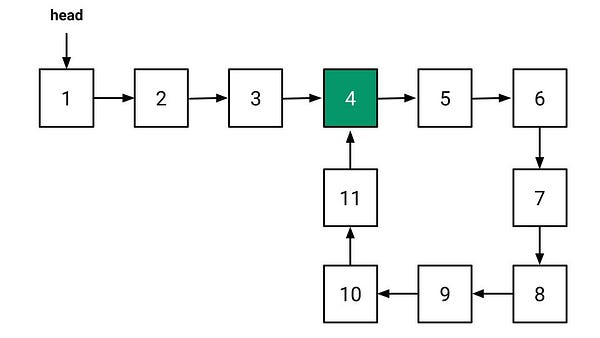Output: true

Explanation: Nodes with values 3 and 11 point to the node with value 3 and form a cycle in the input linked list. So we return true.

Example 2

Input: 2 -> 20 -> 6 -> 19 -> 13 -> 4

Output: false

Explanation: There is no cycle in the linked list. So we return false.

### Discussed solution approaches

•  A brute force approach using a hash table
• Using boolean flag inside linked list node (augmentation)
• An efficient approach  using fast and slow pointers (Floyd’s cycle detection algorithm)

### A brute force approach using a hash table

Solution Idea

The basic idea is to traverse the linked list, store the visited nodes, and If the visited node encounters again during the traversal, then it means there is a cycle. Otherwise, if we reach the end of the linked list or discover a NULL pointer, it means there is no cycle. But how do we store the visited nodes and search their duplicate presence? Think! We can use the hash table because it can perform insert and search operations efficiently in O(1) time complexity on average.

Solution Steps

• Traverse each node of the linked list one by one using a loop and store the node addresses in a Hash Table.
• Now search the current node in the hash table. If the node is already present there or previously visited node encounter again, then return true. Otherwise, insert the node in the hash table and move to the next node.
• When we encounter the NULL pointer during the traversal i.e. head == NULL, we return false. It means: we have reached the end, and there is no cycle in the linked list.

Solution Pseudocode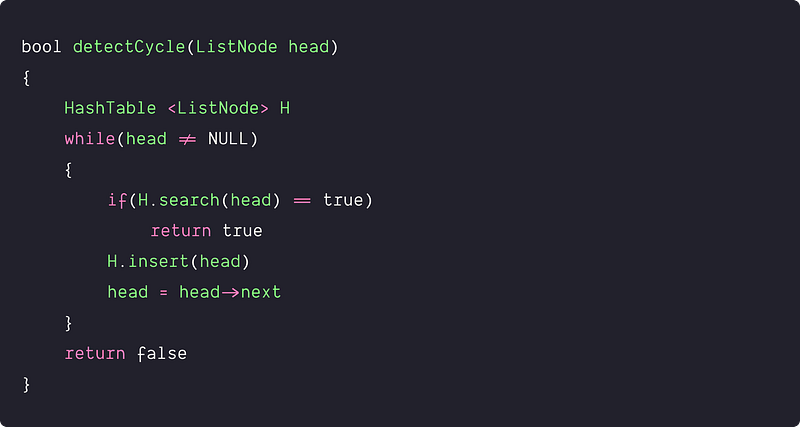Time and space complexity analysis

Only one traversal of the linked list is needed in the above code. In other words, the algorithm is performing insert & search operations only once for each node in the linked list. Time complexity = n*O(1) =O(n). We are using a hash table for storing node addresses, so space complexity = O(n)

### Using boolean flag inside linked list node (augmentation)

Solution Idea

Here we are optimizing the above approach by avoiding the overhead of hash table operations. The idea would be to modify the linked list node structure and add an extra boolean flag in the definition of the linked list node. This could help us mark the visited nodes and identify their duplicate presence, i.e. whenever the node is visited, it is marked 1, and if we encounter the node with a marked flag again, there is a cycle.

Solution Pseudocode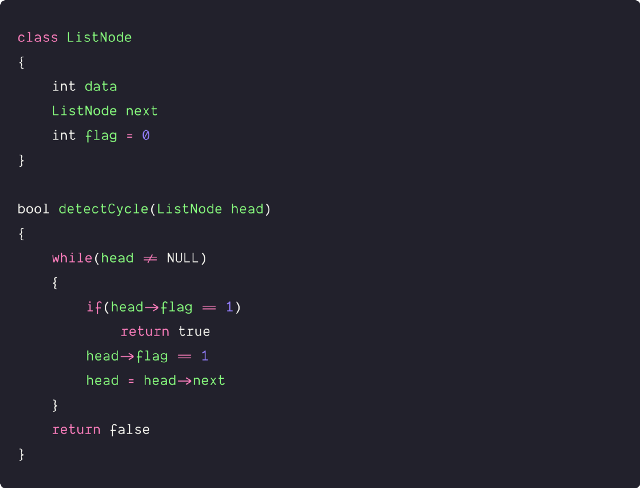Time and space complexity analysis

It is working in O(n) time complexity as the algorithm is traversing each node once. We are using an extra variable with each node, so space complexity = O(n)

### An efficient approach  using Floyd’s cycle detection algorithm

Solution Idea

The above solutions use O(n) space, so the critical question is: can we optimize further and solve it using O(1) space complexity?

The idea of an O(1) space solution is based on Floyd’s cycle-finding algorithm that uses only two pointers at different speeds: a slow pointer and a fast pointer. At each step of the iteration, the slow pointer moves 1 step simultaneously while the fast pointer moves two steps at a time. It is also called the “tortoise and the hare algorithm.”

• Both pointers start from the head node.
• If there is no cycle present in the list, the fast pointer will reach the end.
• But if there is a cycle in the list, then both pointers will meet at some point. But why will this happen? So here is the basic intuition: Suppose two people are moving in a circular track at different speeds, then after some point in time, a fast person will meet the slow person. (Think!)

The above idea works because of the following critical facts:

• If we notice the movements of fast and slow pointers, the distance between them increases by one after every step of the iteration.
• When a slow pointer enters the loop, the fast pointer must be inside the loop. At this point, suppose the fast pointer is distance k from the slow pointer, and the length of the cycle is l.
• From this point, after the 1st iteration, the distance between slow and fast becomes k + 1, after the 2nd iteration, k + 2, and so on. When this distance becomes l, they will meet because they are moving in a cycle of length l. So total steps traveled in the cycle before meeting at some common point = l - k.

Solution Pseudocode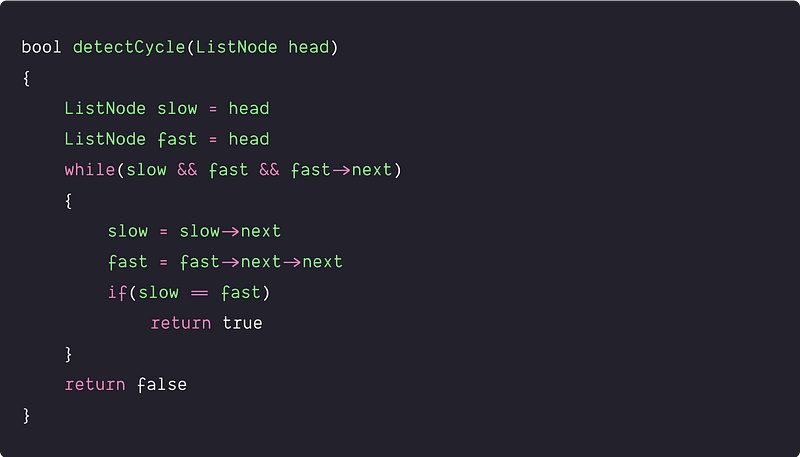Time and space complexity analysis

Suppose for the analysis purpose :

• Total no. of nodes in the linked list = n
• The length of the linked list cycle (if any) = l
• The distance of the starting point of the cycle from the beginning of the list = m. Here l + m = n
• When a slow pointer enters the loop, the fast pointer distance from the slow pointer = k.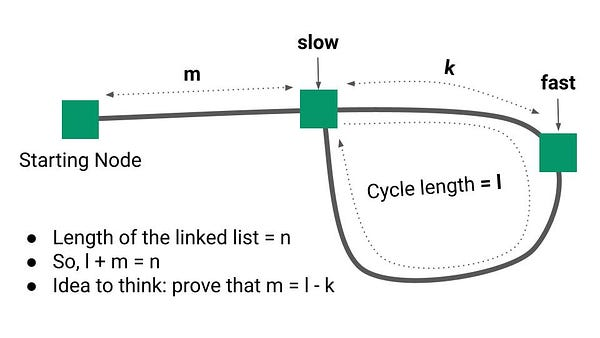Case 1 : When there is no loop in the linked list then the fast pointer will reach the end after n/2 steps. So time complexity = O(n)

Case 1 : When there is a loop in the linked list.

The slow or fast pointer will move m steps in the linked list before the slow pointer enters the loop. Now inside the loop, both pointers will travels (l - k) steps before meeting at some common point. (Think!) So total no of steps traveled by both pointers = m + (l - k) = l + m - k = n - k. So what would be the value of k? Let's think!

If the slow pointer travels m distance from the start to reach the bridge point, then the fast pointer will travel 2m distance from the start. In this situation, there will be two cases:

• If m < l, then k = m and the total no of steps traveled by both pointers = n - m. So time complexity in the worst case = O(n), where the worst-case scenario occurs when m = 1. What would be the best-case scenario? Think!
• If m >= l, then fast pointers will first move m distance to reach the bridge point and revolve in a cycle m/l times. Distance of fast pointer from the bridge point (k) = m mod l and total no of steps traveled by both pointers = n - k = n - m mod l. So time complexity in the worst case = O(n), where the worst-case scenario occurs when m = l. What would be the best-case scenario? Think!

So overall, time complexity would be O(n) in the worst-case. The algorithm is working in constant space, space complexity: O(1)

### Critical ideas to think!

• How do we remove the loop if there is any loop present in the linked list?
• How do we find the length of the loop in the linked list?
• How do we find the bridge node if there is any loop present?
• What would be the value of k when l > m and l < m?
• Can we solve this problem using some other approach?
• Is it necessary to move the fast pointer with a double speed of the slow pointer? What will happen if we move the fast pointer with the speed 4 times of the slow pointer?

### Comparison of time and space complexities

•  Using a hash table: Time = O(n), Space = O(n)
• Using linked list augmentation: Time = O(n), Space = O(n)
• Floyd’s cycle detection algorithm: Time = O(n), Space = O(1)

### Suggested problems to practice

Enjoy learning, Enjoy problem-solving, Enjoy algorithms!

Get well-designed application and interview centirc content on ds-algorithms, machine learning, system design and oops. Content will be delivered weekly.